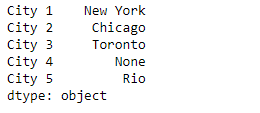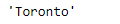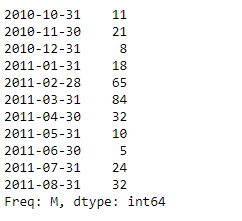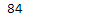# Python | Pandas Series.get()

• Last Updated : 13 Feb, 2019

Pandas series is a One-dimensional ndarray with axis labels. The labels need not be unique but must be a hashable type. The object supports both integer- and label-based indexing and provides a host of methods for performing operations involving the index.

Pandas` Series.get()` function get item from object for given key (DataFrame column, Panel slice, etc.). Returns default value if not found.

Syntax: Series.get(key, default=None)

Parameter :
key : object

Returns : value : same type as items contained in object

Example #1: Use `Series.get()` function to get the value for the passed index label in the given series object.

 `# importing pandas as pd ` `import` `pandas as pd ` ` `  `# Creating the Series ` `sr ``=` `pd.Series([``'New York'``, ``'Chicago'``, ``'Toronto'``, ``None``, ``'Rio'``]) ` ` `  `# Create the Index ` `index_ ``=` `[``'City 1'``, ``'City 2'``, ``'City 3'``, ``'City 4'``, ``'City 5'``]  ` ` `  `# set the index ` `sr.index ``=` `index_ ` ` `  `# Print the series ` `print``(sr) `

Output :Now we will use `Series.get()` function to return the value for the passed index label in the given series object.

 `# return the value corresponding to ` `# the passe index label ` `result ``=` `sr.get(key ``=` `'City 3'``) ` ` `  `# Print the result ` `print``(result) `

Output :As we can see in the output, the `Series.get()` function has returned the value corresponding to the passed index label.

Example #2 : Use `Series.get()` function to get the value for the passed index label in the given series object.

 `# importing pandas as pd ` `import` `pandas as pd ` ` `  `# Creating the Series ` `sr ``=` `pd.Series([``11``, ``21``, ``8``, ``18``, ``65``, ``84``, ``32``, ``10``, ``5``, ``24``, ``32``]) ` ` `  `# Create the Index ` `index_ ``=` `pd.date_range(``'2010-10-09'``, periods ``=` `11``, freq ``=``'M'``) ` ` `  `# set the index ` `sr.index ``=` `index_ ` ` `  `# Print the series ` `print``(sr) `

Output :Now we will use `Series.get()` function to return the value for the passed index label in the given series object.

 `# return the value corresponding to ` `# the passe index label ` `result ``=` `sr.get(key ``=` `'2011-03-31'``) ` ` `  `# Print the result ` `print``(result) `

Output :As we can see in the output, the `Series.get()` function has returned the value corresponding to the passed index label.

My Personal Notes arrow_drop_up
Recommended Articles
Page :